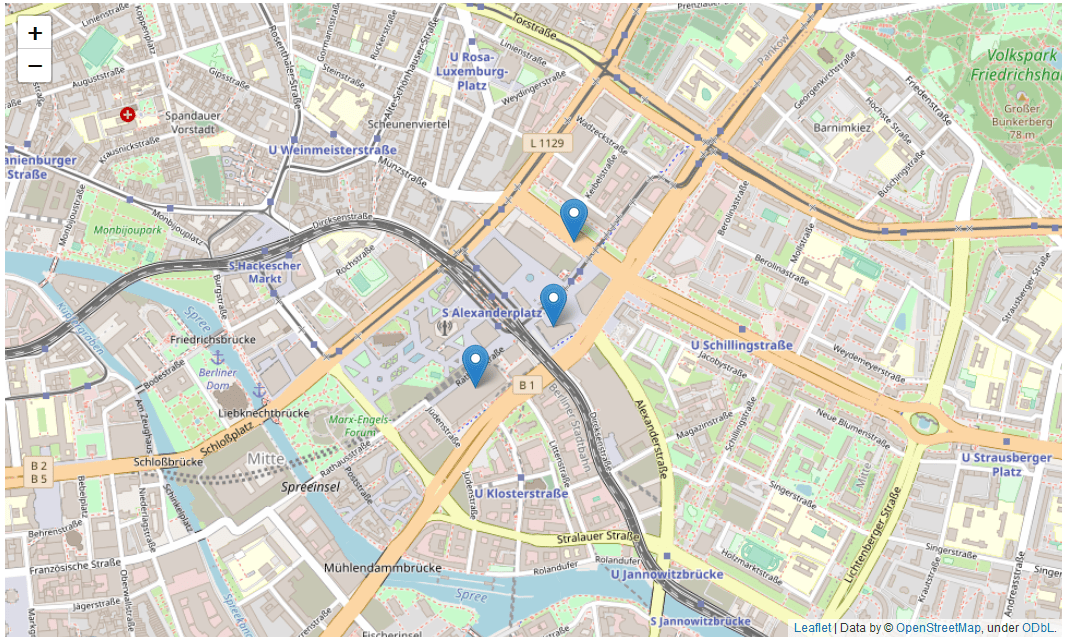# Python中具有Geopy和Folium的多个地理编码地图标记```＃导入熊猫
import pandas
＃读取包含位置数据的csv文件
＃显示csv文件中的表
data
```

`type(data)`
`pandas.core.frame.DataFrame`

```＃将国家，城市和街道合并为一个地址字符串
data["addresses"] = data["country"] + ", " + data["city"] + ", " + data["street "]
＃导入geopy模块
import geopy
＃创建一个服务对象
service = geopy.Nominatim(user_agent = "myGeocoder")
＃对熊猫人DataFrame使用.apply（）方法对每个地址进行地址解析
from geopy.extra.rate_limiter import RateLimiter data["coordinates"] = data["addresses"].apply(RateLimiter(service.geocode,min_delay_seconds=1))
＃显示表格数据```

```type(data["coordinates"])
geopy.location.Location```

`data["coordinates"].longitude`
`13.4144809`
`data["coordinates"].latitude`
`52.5228654`

```＃提取经度和纬度值以分离列表
longs = [coord.longitude for coord in data["coordinates"]]
lats = [coord.latitude for coord in data["coordinates"]]
＃计算平均经度和纬度值
import statistics
meanLong = statistics.mean(longs)
meanLat = statistics.mean(lats)
＃显示结果
print("meanLong = " + str(meanLong) + "; meanLat = " + str(meanLat))```
`meanLong = 13.412910038576356; meanLat = 52.52100943333333`
```[longs,lats]
```
```[[13.4144809, 13.4136431, 13.410606115729072],
[52.5228654, 52.5208149, 52.519348]]```

```＃导入FOLIUM
import folium
＃创建以柏林为中心的基础地图
mapObj = folium.Map(location = [meanLat,meanLong], zoom_start = 15)
＃为柏林创建标记对象，对数据DataFrame中的每个位置一一创建
for i in range(0,data.shape): # .shape for Pandas DataFrame is the number of rows
＃为位置i创建标记
markerObj = folium.Marker(location = [lats[i],longs[i]])
＃在地图上添加标记